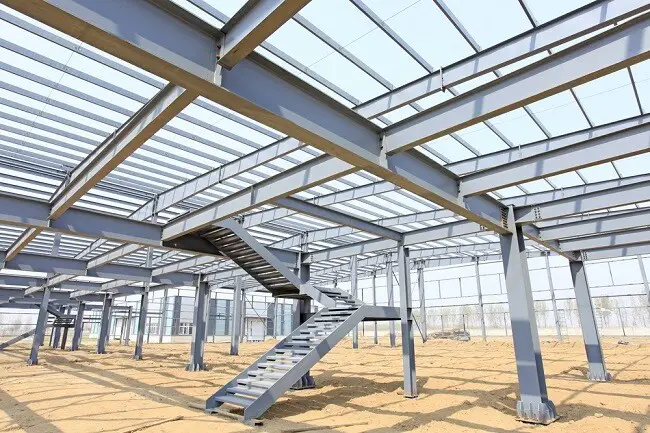# Design Example of Steel Beams According to Eurocode 3Introduction
This post deals with the design of simply supported I-beam section subjected to permanent and variable loads according to Eurocode 3. The design involves selecting the appropriate section that will satisfy limit state requirements.

Problem Statement
It is desired to select an appropriate section to satisfy ultimate and serviceability limit state requirements for a laterally restrained simply supported beam that is subjected to the following loads;
Permanent Load Gk = 38 kN/m
Variable Load Qk = 12 kN/m

The length of the beam = 7.5m

Solution

At ultimate limit state,
PEd = 1.35Gk + 1.5Qk
PEd = 1.35(38) + 1.5(12) = 69.3 kN/m

An advanced UK beam S275 is to be used for this design.
Fy = 275 N/mm2
γm0 = 1.0 (Clause 6.1(1) NA 2.15 BS EN 1993-1- 1:2005)

The required section is supposed to have a plastic modulus about the y-y axis that is greater than;
Wpl,y = My,Edγm0/Fy

Wpl,y = (487.265 × 103 × 1.0)/275 = 1771.872 cm3

From steel tables, try section 457 x 191 x 82        Wpl,y = 1830 cm3

CHECK OUT ALSO….
Design of Steel Beams to BS 5950 – 1: 2000
Structural Analysis of Compound Arch-Frame Structure

Properties
h = 460.0mm; b = 191.3mm; d = 407.6mm; tw = 9.9mm; tf; r = 10.2mm; A = 104 cm4; Iy = 37100 cm4; Iz = 1870 cm4; Wel,y = 1610 cm3; Wel,y = 1830 cm3

hw = h – 2tf = 428.0mm

E (Modulus of elasticity) = 210000 N/mm(Clause 3.2.6(1))

Classification of section
ε = √(235/Fy) = √(235/275) = 0.92 (Table 5.2 BS EN 1993-1- 1:2005)

Outstand flange: flange under uniform compression c = (b – tw – 2r)/2 = [191.3 – 9.9 – 2(10.2)]/2 = 80.5mm

c/tf = 80.5/16.0 = 5.03

The limiting value for class 1 is c/tf  ≤ 9ε = 9 × 0.92
5.03 < 8.28
Therefore, outstand flange in compression is class 1

Internal Compression Part (Web under pure bending)
c = d = 407.6mm
c/tw = 407.9/9.9 = 41.17

The limiting value for class 1 is c/tw ≤ 72ε = 72 × 0.92 = 66.24
41.17 < 66.24
Therefore, the web is plastic. Therefore, the entire section is class 1 plastic.

Member Resistance Verification
Moment Resistance
For the structure under consideration, the maximum bending moment occurs where the shear force is zeo. Therefore, the bending moment does not need to be reduced for the presence of shear force (clause 6.2.8(2))

MEd/Mc,Rd ≤  1.0 (clause 6.2.5(1))

Mc,Rd = Mpl,Rd = (Mpl,y × Fy)/γm0

Mc,Rd = Mpl,Rd = [(1830 × 275)/1.0] × 10-3 = 503 kNm

MEd/Mc,Rd = 487.265/503 = 0.9687 < 1.0 Ok

Shear Resistance (clause 6.6.2)
The basic design requirement is;

VEd/Vc,Rd ≤  1.0

Vc,Rd = Vpl,Rd = Av(F/ √3)/γm0 (for class 1 sections)
For rolled I-section with shear parallel to the web, the shear area is;

Av = A – 2btf + (tw + 2r)tf (for class 1 sections) but not less than ηhwtw

Av = (104 × 102 – (2 × 191.3 × 16) + [9.9 + 2(10.2)] × 16 = 4763 mm2
η = 1.0 (conservative)
ηhwt= (1.0 × 428 × 9.9) = 4237 mm2
4763 > 4237
Therefore, Av = 4763 mm2

The shear resistance is therefore;
Vc,Rd = Vpl,Rd = [4763 × (275 / √3)/1.0]  × 10-3 = 756 kN

VEd/Vc,Rd = 259.875/756 = 0.343 < 1.0 Ok

Shear Buckling
Shear buckling of the unstiffnened web will not need to be considered if;

hw/t≤  72ε/η

hw/t= 428.0/9.9 = 43
72ε/η  = (72 ×  0.92)/1.0  = 66

43 < 66 Therefore shear buckling need not be considered.

Serviceability limit state
Vertical deflections are computed based on variable loads. Permanent loads need not be considered.(BS EN 1993-1-1 NA 2.23)

Qk = 12 kN/m

w = 5ql4/384EI

w = (5 × 12 × 75004)/(384 × 210000 × 37100 × 104) = 6.345mm
Span/360 = 7500/360 = 20.833mm (BS EN 1993-1-1 NA 2.23)

6.345mm < 20.833mm. Therefore, deflection is satisfactory

Thank you for visiting Structville Today.

Thickness design of column base plate connections

Our amazing facebook interactive page can be found at;

1. • Great post. I think it is good for visitors. I like this kind of website where has a lot of real information, It proved to be very helpful. Thanks for admin, His creativity, Presentation, Information and all is good.

2.H

hello,are the udls stated here for a singular beam or the whole structure?
sorry, I’m a student with not much knowledge yet!

3.H

hello,are the udls stated here for a singular beam or the whole structure?
sorry, I’m a student with not much knowledge yet!

4.Fitsum Kifle

i am stranger to this website, i am extremely surprised in your post. i have got some valuable concepts from your post thank you too!

5.Sunil Biradar

Please check for LTB in this the beam is marginally passing so reduction of moment capacity has to be checked and it may fail.

6.engineer kaleab d.

wow nice doing keep it up….